# Cochran-Mantel-Haenszel CMH test in Excel tutorial

2017-10-20

This tutorial will help you run and interpret a Cochran-Mantel-Haenszel CMH test in Excel using XLSTAT.
Not sure this is the statistical test you are looking for? Check out this guide.

## Dataset to run a Cochran-Mantel-Haenszel test and goal of this tutorial

An Excel sheet with both the data and the results can be downloaded by clicking here.

The dataset has first been used in [Mantel N. (1963). Chi-Square Tests with One Degree of Freedom; Extensions of the Mantel- Haenszel Procedure. Journal of the American Statistical Association, 58, 303, 690-700], and corresponds to an experiment in which rabbits were injected with beta-hemolytic streptococci, and then immediately or after 90 minutes with a dose of penicillin varying from 1/8 to 4.

The aim of the experiment is to check first if there is a dependency between the survival or death of the rabbit and the delay in injection of penicillin.

## Setting up a Cochran-Mantel-Haenszel test in Excel with XLSTAT

After opening XLSTAT, click the Non parametric tests button in the ribbon and select Cochran-Mantel-Haenszel test (see below).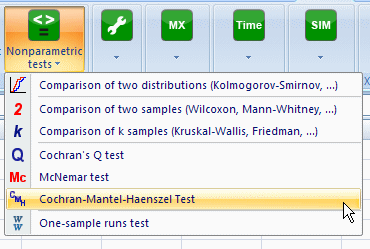Once you've clicked the button, the dialog box appears. You can then select the data on the Excel sheet. Two formats are possible. For illustration the two data formats are presented in the datasheet. However for the tutorial, only the format Contingency Table is used.

You must select at first the 5 contingency tables (each corresponding to a level of penicillin). The option Column label is left enabled as the first line of the selection contains the names of two categories of the response variable (Cured or Died).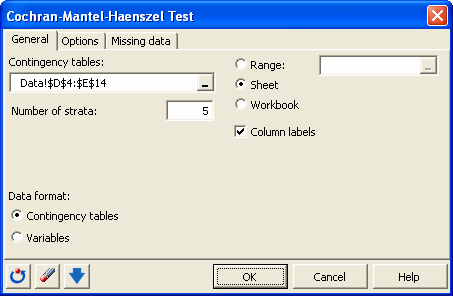In the Options tab, the alternative hypothesis proposed is valid only when the selected tables are 2x2, which is the case here.

The Common odds ratio <> 1 option corresponds to the assumption of dependence. In the case of 2x2 tables it is possible to calculate an exact p-value.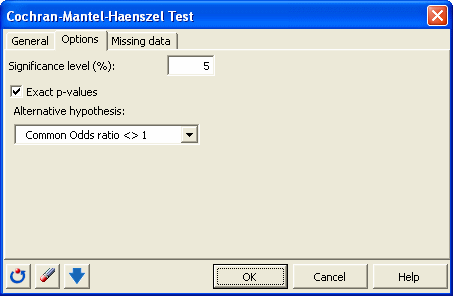Once you have clicked the OK button, the computation starts. The results are then displayed.

## Interpreting the results of a Cochran-Mantel-Haenszel test

XLSTAT first provides a table that displays the Cramer's V, the chi-square statistics and the p-values (exact in the case of 2x2 tables) to evaluate for each sub-table if it corresponds to a case independence or not. These statistics are calculable only if the marginal sums are nonzero in each sub-tables. We see here that only the table corresponding to the dose 1 / 2 is close to dependence.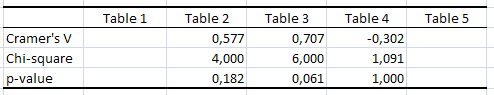The Cramer's V has the advantage of being interpretable in terms of strength of link between variables. In the case of 2x2 tables, it is indeed close to 0 when there is no link, -1 if the cross categories (1 and 2, 2 and 1) are related, and close to 1 when categories with the same index (1 and 1, 2 and 2) are linked.

However, when taking the tables all together for a Cochran-Mantel-Haenszel test, we obtain a common odds ratio of 7, with a 95% confidence interval that does not included the value 1. The exact p-value of 0.04 (we would obtain 0.047 if the exact option was not selected) leads to the conclusion that there is generally a link between the delay and the fact that a rabbit will survive or not the injection of streptococci.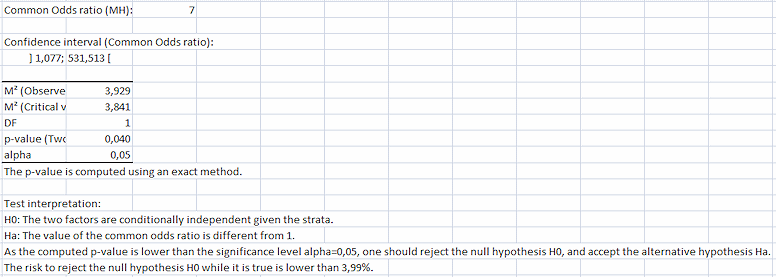This result may seem surprising because none of the sub-tables for which one could calculate the statistics showed a dependency. By combining the tables we show there is a dependence. As the odds ratio is greater than 1, there is a positive effect of an immediate injection of penicillin on the survival.

The Cochran-Mantel-Haenszel should be used with caution. It is particularly not recommended when there is a level 3 interaction between the 3 variables. In our case, this would correspond to the situation where a higher dose of penicillin would increase the impact of delay on the survival. This situation can correspond to an evolution of Cramer's V from a value close to -1 to 1. And despite an obvious strong dependence, the Cochran-Mantel-Haenszel could conclude that there is independence. Hence the interest that the Cramer's V are almost homogeneous when one concludes there is independence.

#### Contact our technical support team: support@xlstat.com

https://cdn.desk.com/
false
desk